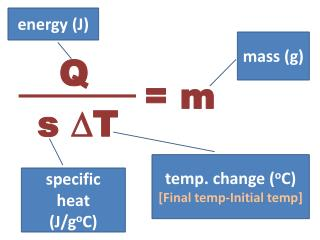DownloadDownload Presentations ∆T

# s ∆T

Télécharger la présentation## s ∆T

- - - - - - - - - - - - - - - - - - - - - - - - - - - E N D - - - - - - - - - - - - - - - - - - - - - - - - - - -
##### Presentation Transcript

1. energy (J) mass (g) Q = m s ∆T temp. change (oC) [Final temp-Initial temp] specific heat (J/goC)

2. A werewolf hunter cools her newly handmade silver bullet from 952 oC to 22 oC in a bucket of water. Calculate the energy change in kcal for the bullet if it has a mass of 35.2 grams. Q = s ∙ m ∙ ∆T Q = (0.240 J/goC) (35.2g) (22oC - 952oC) Q = -7856.64 J -7856.64 J x 1 cal x 1 kcal = -1.88 kcal 4.184 J 1000 cal

3. A 2.6 g sample of a metal requires 15.2 J of energy to change its temperature from 21oC to 34oC. Use the table to determine the identity of metal. s = Q . m∆T s = 15.2 J (2.6g) (34oC - 21oC) s = 0.45 J/goC

4. A sample of gold loses 3.1 J of energy when it cools from 27 oC to 19oC. What is the mass of this sample of gold? m = Q s∆T m = - 3.1 J (0.129 J/goC ) (19oC - 27oC) m = 3 g Au

5. If 294 J of heat is transferred to a 10.0 g sample of silver at 25oC, what is the final temperature of the silver? (sAg = 0.24 J/goC) ∆T = Q . sm Tfinal - 25oC = 294 J (0.24 J/goC ) (10.0g) Tfinal = 150oC